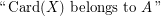# Courcelle, Bruno

## Monadic second-order logic with cardinality predicates ★★

Author(s): Courcelle

The problem concerns the extension of Monadic Second Order Logic (over a binary relation representing the edge relation) with the following atomic formulas:

\item\itemwhereis a fixed recursive set of integers.

Let us fixand a closed formulain this language.

Conjecture   Is it true that the validity offor a graphof tree-width at mostcan be tested in polynomial time in the size of?